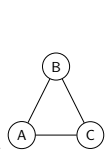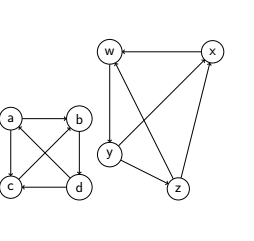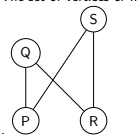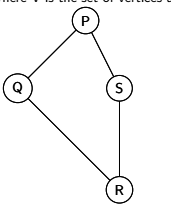# Graph

Graph Theory is the subject of study of graphs, which are mathematical structures used to model pairwise relations between objects.

The paper written by leonhard Euler on “Seven Bridges of Konlgsberg” and published in 1736 as regard as the first paper in the history of graph theory.More than centure after Euler’s paper on “Seven Bridges of Konlgsberg” while listing was introduced then concepet of Topology was introduced.Cayley was let by an intrest in particular analytical forms arising from differential calculus.To study particular case of graphs.In particulat the team graph was introduced by Sylvester in a paper published in 1878.THe first textbook in graph theory was written by Derves Konig,abd was published in 1936.

Graph is simply a pictorial representation of object.It is also defined as the set and set of certaion relation.Mathematically we write graph as G = (V, A). Where V is the set of vertices and A is the set of arcs.## Types of Graph

simply there are two types of graph.These are listed below.

• Directed Graph
• undirected Graph

## 1. Directed Graph

If all arcs are orented from one particular vertex to the another particular vertex then graph becomes a directed graph.It is also defined as if there is direction are given to the arc of the graph then graph becomes directed graph.Directed graph is also written as digraph.following are the some figure where direction is given to the arcs.There are some terminology related to the digraph.

• Tail

#### 1.Tail

The vertex from which the arc begin is called tail of the arc.

The vertex at which the arc end is called head of arcs.

If the arc share the common end node then this type of arc is called adjacency arc.In above figure 1 {a,b,c,d} are vertices {(a, b),(b, d),(d, c)(a,c),(b, d)} are arcs.Head set of figure 1 are (b, d, c, b, a) and tail set of figure 1 are (a, b, d, c, a)

## 2.Undirected Graph

A graph having no direction in between any vertex is called undirected graph.It is called simply a graph.In case of undirected graph we can take any vertex as a first vertex and any vertex as the second vertex.Some figure are given below.Tags:

Categories:

Updated: